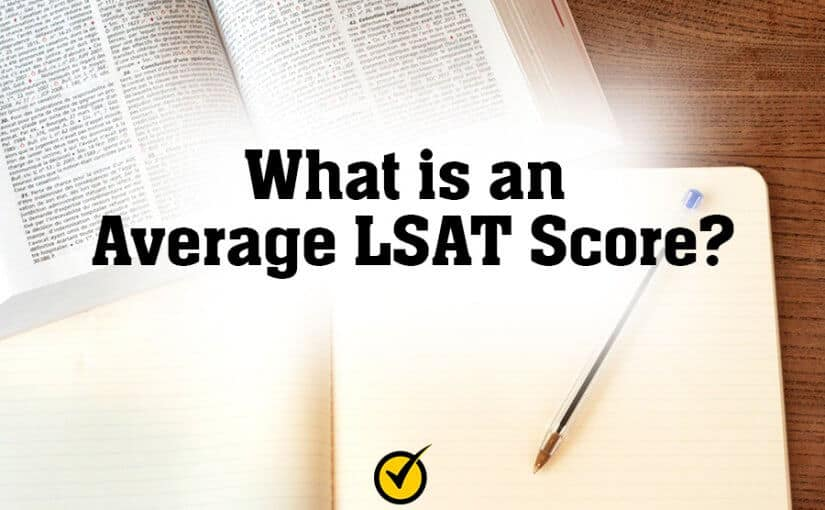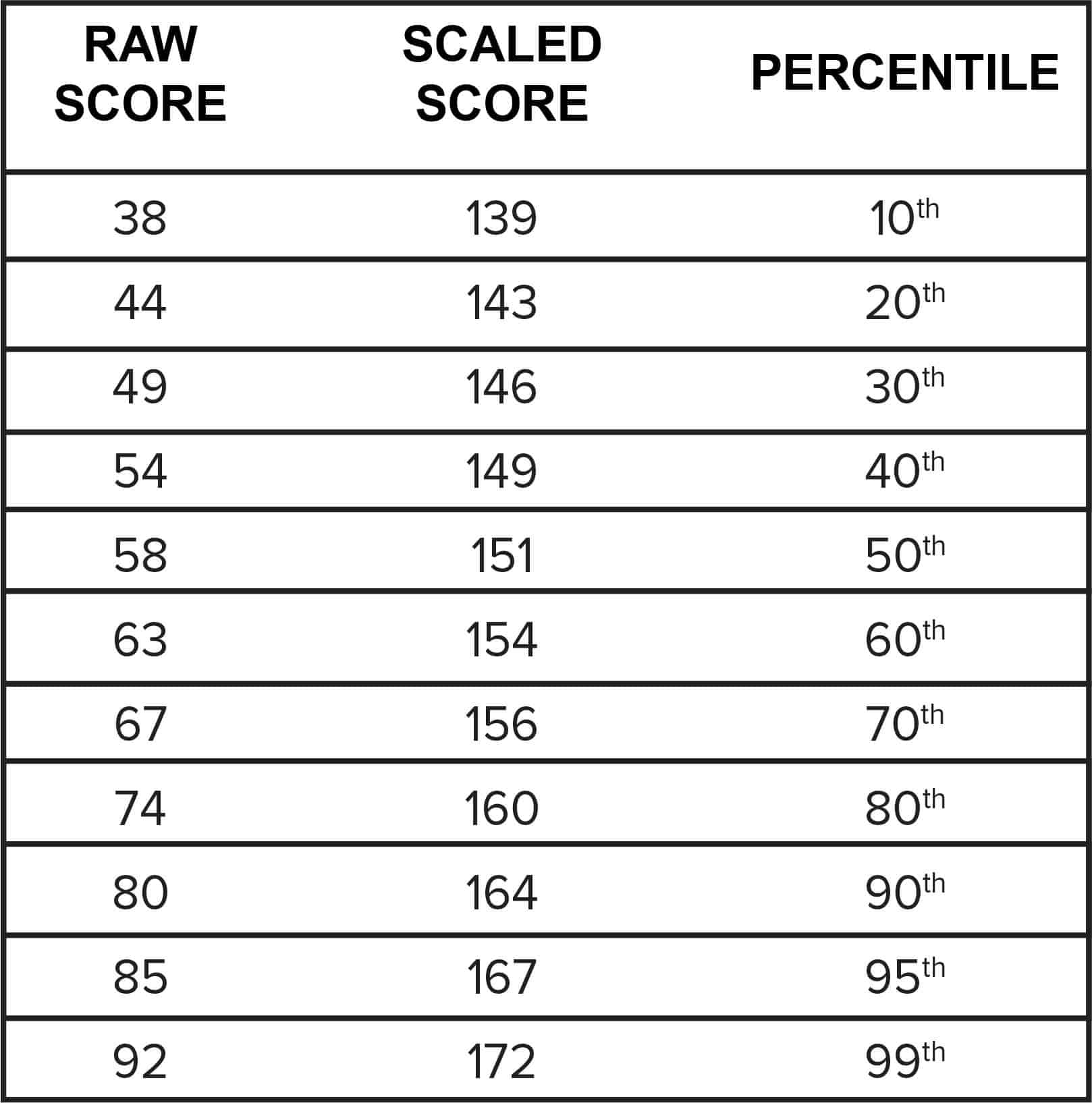# What is an Average LSAT Score?

You know that getting a good LSAT score can help you get into the law school of your choice. The score that you receive on the LSAT is the most important factor that determines your admittance to law school.

How Long is the LSAT?

## Understanding How the LSAT is Scored

Each section of the LSAT contains a different amount of questions but each section will be scored basically the same. The raw score that you receive is the number of questions that you answer correctly out of the total number of questions that are on the exam. There are no point deductions for incorrect answers. For example, if you receive a raw score of 45, that means that you answered 45 questions correctly. The LSAT contains between 94 to 106 questions which can give you a raw score between 0 to 94 or 106 (depending on the number of questions on the exam.)

Analytical Reasoning Practice Test

After your raw score, you’ll receive a scaled score. The raw score is converted into a scaled score that ranges between 120 to 180. The scaled score is the score that law schools look at for their admission decisions.

LSAT Practice Test

You’ll also receive a percentile score. The percentile score shows you how well you’ve done on the exam compared with others that have taken the exam over a three year period.## What is the Average LSAT Score?The LSAT is scored on a range of 120 to 180 with 180 being the highest possible score. The average LSAT score is around 152 which will put you right in the middle. This means that you’ll need to get about 60 out of 94 to 106 questions right to get the average score.